# gallery

## 语法

``[A1,A2,...,Am] = gallery(matrixname,P1,P2,...,Pn)``
``[A1,A2,...,Am] = gallery(matrixname,P1,P2,...,Pn,typename)``
``A = gallery(3)``
``A = gallery(5)``

## 说明

``[A1,A2,...,Am] = gallery(matrixname,P1,P2,...,Pn)` 生成由 `matrixname` 指定的一系列测试矩阵。`P1,P2,...,Pn` 是单个矩阵系列要求的输入参数。调用语法中使用的输入参数 `P1,P2,...,Pn` 的数目因矩阵而异。`matrixname` 一节中说明了每个矩阵系列的确切调用语法。`
``[A1,A2,...,Am] = gallery(matrixname,P1,P2,...,Pn,typename)` 还指定生成的测试矩阵的数据类型。`

``A = gallery(3)` 生成一个对扰动敏感的病态 3×3 矩阵。`

``A = gallery(5)` 生成一个 5×5 矩阵，它具有一个有趣的特征值问题，即对舍入误差很敏感。`

## 示例

`C = gallery('circul',11);`

```imagesc(C) axis square colorbar```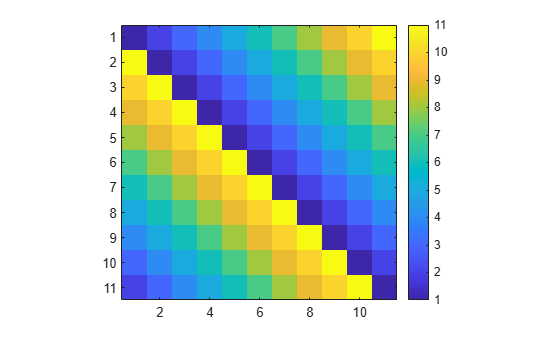`G = gallery('grcar',11);`

```imagesc(G) axis square colorbar```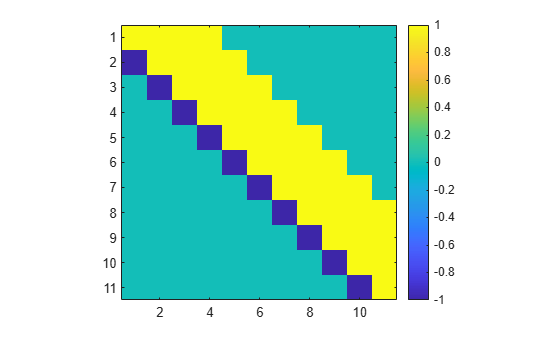`M = gallery('minij',11);`

```imagesc(M) axis square colorbar```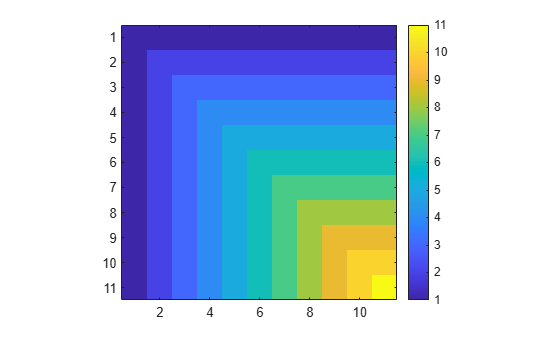`A = gallery('dramadah',6)`
```A = 6×6 1 1 0 1 0 0 0 1 1 0 1 0 0 0 1 1 0 1 1 0 0 1 1 0 1 1 0 0 1 1 0 1 1 0 0 1 ```
`detA = det(A)`
```detA = -1 ```
`invA = inv(A)`
```invA = 6×6 -1 -2 -3 4 -2 5 1 1 1 -2 1 -2 -1 -1 -2 3 -2 4 1 1 2 -2 1 -3 0 1 1 -1 1 -2 0 0 1 -1 1 -1 ```

```rng('default') A = randn(6,3)```
```A = 6×3 0.5377 -0.4336 0.7254 1.8339 0.3426 -0.0631 -2.2588 3.5784 0.7147 0.8622 2.7694 -0.2050 0.3188 -1.3499 -0.1241 -1.3077 3.0349 1.4897 ```

```[v1,beta1] = gallery('house',A(:,1)); P1 = eye(6) - beta1*(v1*v1'); A1 = P1*A```
```A1 = 6×3 -3.3630 2.8841 1.0421 -0.0000 1.9024 0.0858 0 1.6571 0.5314 0 3.5028 -0.1350 -0.0000 -1.0788 -0.0983 0.0000 1.9227 1.3835 ```

```[v2,beta2] = gallery('house',A1(2:end,2)); v2 = [0;v2]; P2 = eye(6) - beta2*(v2*v2'); A2 = P2*A1```
```A2 = 6×3 -3.3630 2.8841 1.0421 -0.0000 -4.8472 -0.6885 0.0000 0 0.3413 0.0000 -0.0000 -0.5368 -0.0000 0.0000 0.0255 0.0000 0.0000 1.1630 ```

```[v3,beta3] = gallery('house',A2(3:end,3)); v3 = [0;0;v3]; P3 = eye(6) - beta3*(v3*v3'); R = P3*A2```
```R = 6×3 -3.3630 2.8841 1.0421 -0.0000 -4.8472 -0.6885 -0.0000 -0.0000 -1.3258 0.0000 -0.0000 0.0000 -0.0000 0.0000 0.0000 0.0000 -0.0000 0.0000 ```

`Q = P1*P2*P3`
```Q = 6×6 -0.1599 -0.0057 -0.6699 0.4983 -0.2036 -0.4857 -0.5453 -0.3952 -0.1759 -0.6432 0.1342 -0.2895 0.6717 -0.3386 0.1647 -0.0991 0.1551 -0.6109 -0.2564 -0.7239 0.3290 0.5244 0.0805 0.1434 -0.0948 0.2221 -0.0962 0.1872 0.9463 -0.0433 0.3888 -0.3948 -0.6130 -0.1346 0.1203 0.5335 ```

`[Qa,Ra] = qr(A)`
```Qa = 6×6 -0.1599 -0.0057 -0.6699 0.4983 -0.2036 -0.4857 -0.5453 -0.3952 -0.1759 -0.6432 0.1342 -0.2895 0.6717 -0.3386 0.1647 -0.0991 0.1551 -0.6109 -0.2564 -0.7239 0.3290 0.5244 0.0805 0.1434 -0.0948 0.2221 -0.0962 0.1872 0.9463 -0.0433 0.3888 -0.3948 -0.6130 -0.1346 0.1203 0.5335 ```
```Ra = 6×3 -3.3630 2.8841 1.0421 0 -4.8472 -0.6885 0 0 -1.3258 0 0 0 0 0 0 0 0 0 ```

`norm(A - Q*R)`
```ans = 9.0996e-16 ```

`E = zeros(18,20000);`

• 创建一个 1×18 行向量 `x`，其中包含的随机元素为 –0.4 或 0.4。

• 使用向量 `x` 作为输入，创建随机循环矩阵 `A`

• 找出 `A` 的特征值，并将其存储在 `E` 中。

```rng('default') for i = 1:20000 x = -0.4 + 0.8*randi([0 1],1,18); A = gallery('circul',x); E(:,i) = eig(A); end```

```scatter(real(E(:)),imag(E(:)),'b.') xlabel('Re(E)') ylabel('Im(E)') xlim([-3 3]) ylim([-3 3]) axis square```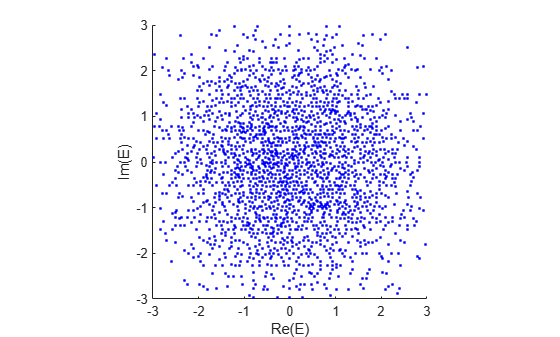`A = gallery(3)`
```A = 3×3 -149 -50 -154 537 180 546 -27 -9 -25 ```

`e = eig(A)`
```e = 3×1 1.0000 2.0000 3.0000 ```

`c = condeig(A)`
```c = 3×1 603.6390 395.2366 219.2920 ```

```rng('default') Ap = A + 1e-3*rand(3)```
```Ap = 3×3 -148.9992 -49.9991 -153.9997 537.0009 180.0006 546.0005 -26.9999 -8.9999 -24.9990 ```

`ep = eig(Ap)`
```ep = 3×1 0.7399 2.1437 3.1188 ```

`delta = ep - e`
```delta = 3×1 -0.2601 0.1437 0.1188 ```

`delta_upper = 1e-3*c`
```delta_upper = 3×1 0.6036 0.3952 0.2193 ```

`A = gallery(5)`
```A = 5×5 -9 11 -21 63 -252 70 -69 141 -421 1684 -575 575 -1149 3451 -13801 3891 -3891 7782 -23345 93365 1024 -1024 2048 -6144 24572 ```

`Afifth = A^5`
```Afifth = 5×5 0 0 0 0 0 0 0 0 0 0 0 0 0 0 0 0 0 0 0 0 0 0 0 0 0 ```

`e = eig(A)`
```e = 5×1 complex -0.0347 + 0.0258i -0.0347 - 0.0258i 0.0138 + 0.0401i 0.0138 - 0.0401i 0.0419 + 0.0000i ```

```plot(0,0,'bo',real(e),imag(e),'r*') axis([-0.1 0.1 -0.1 0.1]) axis square```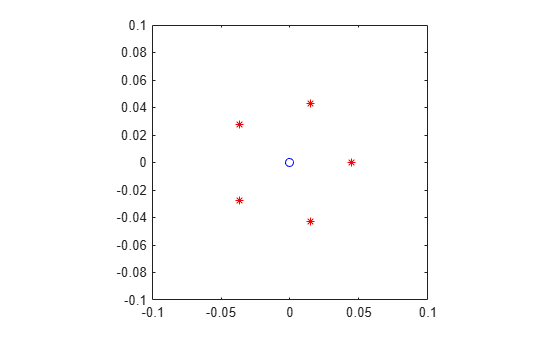```E = zeros(20,5); rng('default') for i = 1:20 E(i,:) = eig(A + eps*randn(5).*A); end plot(0,0,'bo',real(e),imag(e),'r*',real(E),imag(E),'k.') axis([-0.1 0.1 -0.1 0.1]) axis square```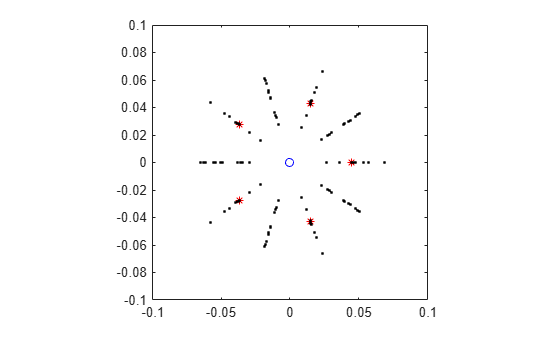## 输入参数

`'binomial'`

• `A = gallery('binomial',n)` 返回一个 `n`×`n` 矩阵，其中包含整数项，满足 `A^2 = 2^(n-1)*eye(n)`

• 矩阵 `B = A*2^((1-n)/2)` 是对合矩阵（矩阵与其逆矩阵相同）。

`'cauchy'`

• `A = gallery('cauchy',x,y)` 返回一个 `n`×`n` 矩阵，其中包含的项满足 `A(i,j) = 1/(x(i)+y(j))`。参数 `x``y` 是长度为 `n` 的向量。如果您为 `x``y` 传入标量，它们将解释为向量 `1:x``1:y`

• `A = gallery('cauchy',x)` 返回与上述 `y = x` 的情况相同的结果。换言之，`A(i,j) = 1/(x(i)+x(j))`

• 柯西矩阵的逆矩阵和行列式的显式公式是已知的。

• 如果 `x``y` 均具有不同元素，则行列式 `det(C)` 为非零值。

• 如果 `0 < x(1) < ... < x(n)``0 < y(1) < ... < y(n)`，则 `C` 为完全正值。

`'chebspec'`

• `A = gallery('chebspec',n,k)` 返回一个大小为 `n`×`n` 的切比雪夫谱微分矩阵。参数 `k` 可以取值 `0`（默认值）或 `1`，它决定输出矩阵的字符。

对于 `k = 0`（无边界条件），`A` 是幂零矩阵，这意味着存在满足 `A^c = 0` 的正整数 `c`。矩阵 `A` 具有空向量 `ones(n,1)`

对于 `k = 1``A` 是非奇异且条件设置良好的矩阵，其特征值具有负实部。

• 对于 `k = 0`，矩阵 `A` 与具有特征值零、大小为 `n` 的 Jordan 块类似。对于两个矩阵 `A``B`，如果存在一个具有相同大小的可逆矩阵 `P` 满足 `B = inv(P)*A*P`，则将这两个矩阵称为相似矩阵。

• 对于这两种 `k` 值，切比雪夫谱微分矩阵的特征向量矩阵是病态的。

`'chebvand'`

• `A = gallery('chebvand',x)` 基于由点 `x` 组成的向量生成原始切比雪夫范德蒙矩阵，这些点定义计算切比雪夫多项式的位置。参数 `x` 是长度为 `n` 的向量，`A` 的大小为 `n`×`n``A` 中的项满足 `A`(`i`,`j`) = T`i` – 1(`x`(`j`))，其中 T`i` – 1`i` - 1 次第一类切比雪夫多项式。如果 `x` 为标量，则将使用区间 `[0,1]` 上的 `x` 个等间距点计算 `A`

• `A = gallery('chebvand',m,x)` 生成上述矩阵的矩形版本（具有 `m` 行），其中 `m` 为标量。

`'chow'`

• `A = gallery('chow',n,alpha,delta)` 返回一个阶数为 `n` 的 Chow 矩阵，它是 `n`×`n` 的下 Hessenberg 矩阵。矩阵 `A` 定义为

`$A={H}_{\alpha }+\text{​}\delta I$`

其中 Hα 的矩阵元素为 Hα(i,j) = α(i – j + 1)（在 (i – j + 1) ≥ 0 时），δ 是系数，I 是 `n`×`n` 单位矩阵。

• `A = gallery('chow',n)` 分别对 `alpha``delta` 使用默认值 `1``0`

• Hα 具有等于零的 `p = floor(n/2)` 特征值。其余特征值等于 `4*alpha*cos(k*pi/(n+2))^2`，其中 `k = 1:(n-p)`

`'circul'`

• `A = gallery('circul',v)` 返回一个 `n`×`n` 循环矩阵，其第一行是长度为 `n` 的向量 `v`。循环矩阵是一种特殊的托普利茨矩阵，其中每行都通过周期性地将上一行中的各元向右移一位来获得。如果 `v` 是标量，则 `A = gallery('circul',1:v)`

• `A` 的特征系统是显式可知的。如果 `t``n` 次单位根，则 `v``w = [1 t t^2 ... t^(n – 1)]` 的内积是 `A` 的特征值，`w(n:-1:1)` 是特征向量。

`'clement'`

• `A = gallery('clement',n,k)` 返回一个主对角线元为 0 的 `n`×`n` 三对角矩阵。对于 `k = 0`（默认值），`A` 是非对称的。对于 `k = 1``A` 是对称的。

• `A = gallery('clement',n,1)` 在对角线方面类似于 `B = gallery('clement',n,0)`，其中存在一个具有相同大小的对角线矩阵 `D` 满足 `B = inv(D)*A*D`

• 如果 `n` 是奇数，则 `A` 是奇异矩阵。

• `A` 的特征值是显式可知的，其中包括正负数字 `n-1``n-3``n-5``...``1``0`

• 前两个属性也适用于 `gallery('tridiag',x,y,z)`，其中 `y = zeros(n,1)`。如果输入向量 `y` 的长度或 `n` 是奇数，则 `gallery('tridiag',x,y,z)` 是奇异的。特征值仍然以正负对组形式出现，但它们并不是显式可知的。

• 对于奇数 `n = 2*m+1``k = 0``gallery('clement',n,0)``m+1` 个奇异值等于 `sqrt((2*m+1)^2 - (2*t+1).^2)`（对于 `t = 0:m`）。

`'compar'`

• `A = gallery('compar',B,k)` 返回 `B` 的比较矩阵。

对于 `k = 0`（默认值），如果 `i == j`，则 `A(i,j) = abs(B(i,j))`，否则 `A(i,j) = -abs(B(i,j))`

对于 `k = 1``A = gallery('compar',B,1)``B` 的每个对角线元素替换为其绝对值，并将每个非对角线元素替换为同一行中非对角线元素的最大绝对值的负值。

• 如果 `B` 是三角矩阵，则 `A = gallery('compar',B,1)` 也是三角矩阵。

`'condex'`

• `A = gallery('condex',n,k,alpha)` 返回条件数估计量的反例矩阵。它采用标量参数 `alpha`（默认值为 100），并返回大小为 `n`×`n` 的矩阵。

该矩阵、其实际大小及其适用的估计量是由 `k` 指定的：

 `k = 1` 4×4 LINPACK 的 RCOND 的反例 `k = 2` 3×3 LINPACK 的 RCOND 的反例 `k = 3` 任意 LINPACK 的 RCOND 的反例（独立于 `alpha`） `k = 4`（默认值） `n >= 4` LAPACK 的 RCOND 的反例（它是作为反例的该矩阵的逆矩阵）

如果 `n` 不等于该矩阵的实际大小，则为该矩阵补上单位矩阵以构成 `n` 阶。

`'cycol'`

• `A = gallery('cycol',n,k)` 返回具有周期性重复列的 `n`×`n` 矩阵，其中一个周期由 `randn(n,k)` 定义的列构成。因此，矩阵 `A` 的秩不能超过 `k``k` 必须是标量。参数 `k` 是标量，默认值为 `round(n/4)`，它不需要整除 `n`

• `A = gallery('cycol',[m n],k)` 返回具有周期性重复列的 `m`×`n` 矩阵，其中一个周期由 `randn(m,k)` 定义的列构成。

`'dorr'`

• `A = gallery('dorr',n,theta)` 返回 Dorr 矩阵，即 `n`×`n` 的行对角占优的三对角矩阵。如果 `theta` 为较小的非负值，则该矩阵是病态的。`theta` 的默认值是 `0.01`

• `[v1,v2,v3] = gallery('dorr',n,alpha)` 返回定义 Dorr 矩阵的向量。Dorr 矩阵本身与 `gallery('tridiag',v1,v2,v3)` 相同。

`'dramadah'`

• `A = gallery('dramadah',n,k)` 返回由 `0``1` 组成的 `n`×`n` 矩阵。`n``k` 必须均为标量。对于 `k = 0``1``mu(A) = norm(inv(A),'fro')` 相对较大（尽管不一定最大）。参数 `k` 确定输出矩阵的字符，如下所示。

 `k = 1`（默认值） `A` 是满足 `abs(det(A)) = 1` 和 `mu(A) > c*(1.75)^2n` 的托普利茨和幺模矩阵，其中 `c` 是常量。`A` 的逆矩阵具有整数项。 `k = 2 ` `A` 是上三角托普利茨矩阵。`A` 的逆矩阵具有整数项。 `k = 3 ` `A` 是托普利茨矩阵，它在下 Hessenberg 二值矩阵（所有元素均为 0 或 1）中具有最大行列式。`det(A)` 等于第 `n` 个斐波那契数。特征值在复平面中的分布很有意义。

`'fiedler'`

• `A = gallery('fiedler',x)`，其中 `x` 是长度为 `n` 的向量，返回含有元素 `A(i,j) = abs(x(i)-x(j))``n`×`n` 对称矩阵。对于标量 `x``A = gallery('fiedler',1:x)`

• 矩阵 `A` 具有占优正特征值，所有其他特征值是负数。

• `inv(A)``det(A)` 的显式公式由 Fiedler 在其著作  中给出。这些公式说明，除了非零 `(1,n)``(n,1)` 元素外，`inv(A)` 是三对角矩阵。

`'forsythe'`

• `A = gallery('forsythe',n,alpha,lambda)` 返回具有特征值 `lambda` 的等于 Jordan 块的 `n`×`n` 矩阵，但 `A(n,1) = alpha` 除外。标量 `alpha``lambda` 的默认值分别为 `sqrt(eps)``0`

• `A` 的特征多项式由 `det(A-t*I) = (lambda-t)^n - alpha*(-1)^n` 给出。

`'frank'`

• 对于默认值 `k = 0``A = gallery('frank',n,k)` 返回大小为 `n`×`n` 的 Frank 矩阵。Frank 矩阵是具有行列式 `1` 的上 Hessenberg 矩阵。如果 `k = 1`，则围绕反对角线 `(1,n)``(2,n-1)`、…、`(n,1)` 反射元素。

• 可以按 Hermite 多项式的零值形式获取 `A` 的特征值。它们是正值并以可逆对组形式出现。

• 如果 `n` 是奇数，则 `1` 是特征值。

• 最小的一半特征值（即最小的 `floor(n/2)` 个特征值）是病态的。

`'gcdmat'`

• `A = gallery('gcdmat',n)` 返回 `n`×`n` 矩阵，其 `A(i,j)` 等于 `gcd(i,j)`

• 对于所有非负 `r`，矩阵 `A` 是对称正定矩阵，`A.^r` 是对称半正定矩阵。

`'gearmat'`

• `A = gallery('gearmat',n,i,j)` 返回 `n`×`n` 矩阵，其中下对角线和上对角线上为 `1``(1,abs(i))` 位置为 `sign(i)``(n,n+1-abs(j))` 位置为 `sign(j)`，其余所有位置为 `0`。参数 `i``j` 的默认值分别为 `n``-n`。它们必须为 `-n``n` 范围内的整数。

• 矩阵 `A` 是奇异矩阵，可具有双重和三重特征值，并且可以是亏损矩阵。

• 所有特征值的形式均为 2*cos(a)，特征向量的形式为 [sin(w+a), sin(w+2a), …, sin(w+na)]，其中 a 和 w 在  中给出。

`'grcar'`

• `A = gallery('grcar',n,k)` 返回 `n`×`n` 托普利茨矩阵，其中下对角线上的元素为 `-1`，主对角线上的元素为 `1`，主对角线上方的 `k` 个对角线上的元素为 `1``k` 必须为整数，默认值为 `k = 3``A` 具有对扰动敏感的特征值。

`'hanowa'`

• `A = gallery('hanowa',n,alpha)` 返回 `2`×`2` 分块矩阵，其中包含四个 `n/2`×`n/2` 分块，形式如下：

```[alpha*eye(n/2) -diag(1:n/2) diag(1:n/2) alpha*eye(n/2)]```

参数 `n` 必须为偶数整数。`alpha` 的默认值为 `-1``A` 具有复数特征值，形式为 `alpha` ± `k*i` (`1 <= k <= n/2`)。

`'house'`

• `[v,beta] = gallery('house',x)` 接受标量或 `n` 元素列向量形式的 `x` 并返回 `beta``v`，以使 `H = eye(n) - beta*v*v'` 为 Householder 矩阵。Householder 矩阵 `H` 满足以下属性

`H*x = -sign(x(1))*norm(x)*e1 `

其中，`e1``eye(n)` 的第一列。

请注意，如果 `x` 是复数，则 `sign(x) = exp(i*arg(x))`，它在 `x` 为非零值时等于 `x./abs(x)`。如果 `x` 为零，则 `v` 为零且 `beta = 1`

• `[v,beta,s] = gallery('house',x,k)` 接受 `n` 元素列向量 `x` 并返回 `v``beta`，以使 `H*x = s*e1`。在此表达式中，`e1``eye(n)` 的第一列，`abs(s) = norm(x)``H = eye(n) - beta*v*v'` 是 Householder 矩阵。

`k` 确定 `s` 的符号，如下所示。

 `k = 0`（默认值） `sign(s) = -sign(x(1))` `k = 1` `sign(s) = sign(x(1))` `k = 2` `sign(s) = 1`（`x` 必须为实数）

如果 `x` 为零，或如果 `x = alpha*e1` (`alpha >= 0`) 且 `k = 1``k = 2`，则 `v` 为零，`beta = 1``s = x(1)`。在本例中，`H = eye(n)` 是单位矩阵，该矩阵并不是严格意义上的 Householder 矩阵。

`'integerdata'`

• `A = gallery('integerdata',imax,[m,n,...],k)` 返回一个 `m`×`n`×... 数组 `A`，其值是均匀分布的整数 `1:imax` 上的一个样本。`k` 是随机种子且必须是区间 `[0,2^32-1]` 中的整数值。使用 `k` 的不同值调用 `gallery('integerdata',...)` 会返回不同数组。使用相同的 `imax`、大小向量和 `k` 重复调用 `gallery('integerdata',...)` 会始终返回同一数组。

在对 `gallery('integerdata',...)` 的任意调用中，您可以用各输入 `m,n,...` 替换大小向量输入 `[m,n,...]`。例如，`gallery('integerdata',7,[1,2,3,4],5)` 等同于 `gallery('integerdata',7,1,2,3,4,5)`

• `A = gallery('integerdata',[imin imax],[m,n,...],k)` 返回一个 `m`×`n`×... 数组 `A`，其值是整数 `imin:imax` 上均匀分布的一个样本。

• `[A1,A2,...,Am] = gallery('integerdata',[imin imax],[m,n,...],k)` 返回包含不同值的多个 `m`×`n`×... 数组 `A1``A2`、...、`Am`

• `[A1,A2,...,Am] = gallery('integerdata',[imin imax],[m,n,...],k,typename)` 生成包含 `typename` 类型的元素的数组。`typename` 必须为 `'double'`（默认值）、`'single'``'uint8'``'uint16'``'uint32'``'int8'``'int16'``int32'`

`'invhess'`

• `A = gallery('invhess',x,y)`，其中 `x` 是长度为 `n` 的向量，`y` 是长度为 `n-1` 的向量。如果 `i <= j`，它返回包含元素 `A(i,j) = x(j)` 的矩阵，否则返回包含元素 `A(i,j) = y(i)` 的矩阵。`A` 的下三角基于向量 `x`，而严格上三角基于 `y`。参数 `y` 默认为 `-x(1:n-1)`

对于标量 `n``gallery('invhess',n)``gallery('invhess',1:n)` 相同。

• 如果对于所有 `i``x(1) ~= 0``x(i+1) ~= y(i)`，则矩阵 `A` 是非奇异矩阵。

• `A` 的逆矩阵是上 Hessenberg 矩阵。

`'invol'`

• `A = gallery('invol',n)` 返回 `n`×`n` 对合矩阵，其中 `A*A = eye(n)``A` 为病态。它是 `hilb(n)` 的沿对角线缩放的版本。

• 矩阵 `B = (eye(n)-A)/2``B = (eye(n)+A)/2` 是幂等的，其中 `B*B = B`

`'ipjfact'`

• `[A,beta] = gallery('ipjfact',n,k)` 返回 `n`×`n`汉克尔矩阵 `A` 及其行列式 `beta`，该行列式是显式可知的。`A` 的逆矩阵也是显式可知的。

如果 `k = 0`（默认值），则 `A` 的元素是 `A(i,j) = factorial(i+j)`。如果 `k = 1`，则 `A` 的元素为 `A(i,j) = 1/factorial(i+j)`

`'jordbloc'`

• `A = gallery('jordbloc',n,lambda)` 返回特征值为 `lambda``n`×`n` Jordan 块。`lambda` 的默认值是 `1`

`'kahan'`

• `A = gallery('kahan',n,theta,pert)` 返回一个上梯形矩阵，该矩阵具有有关条件和秩的估计值的有意义属性。例如，Kahan 矩阵说明使用列置换的 QR 分解无法给出矩阵的良好秩估计。

如果 `n` 是一个二元素向量，则 `A``n(1)`×`n(2)`；否则，`A``n`×`n``theta` 的有用范围是 `0 < theta < pi`，默认值为 `1.2`

为确保使用列主元消去法的 QR 分解不会在存在舍入误差时交换列，将通过 `pert*eps*diag([n:-1:1])` 扰动主对角线。`pert` 的默认值为 `1e3`，此值可确保在 IEEE® 算术运算中，`gallery('kahan',n)` 至少在 `n = 100` 之前不会发生列互换（使用默认值 `theta = 1.2` 时）。

`'kms'`

• `A = gallery('kms',n,rho)` 返回 `n`×`n` Kac-Murdock-Szegö 托普利茨矩阵以使 `A(i,j) = rho^(abs(i-j))`（对于实数 `rho`）。对于复数 `rho`，同一公式也适用，只不过对角线下的元素是共轭的。`rho` 的默认值为 0.5。

• 存在 `A` 的 LDL 分解，其中 `L = inv(gallery('triw',n,-rho,1))'``D = (1-abs(rho)^2)*eye(n)`，但 `D` 的第一个元素 `D(1,1) = 1` 除外。

• 当且仅当 `0 < abs(rho) < 1``A` 为正定矩阵。

• `inv(A)` 的逆矩阵是三对角矩阵。

`'krylov'`

• `A = gallery('krylov',B,x,k)` 返回 Krylov 矩阵，其中 `B``n`×`n` 矩阵，`x` 是长度为 `n` 的向量，`k` 必须为整数。Krylov 矩阵等于

`[x, B*x, B^2*x, ..., B^(k-1)*x]`

（对于列向量 `x`。）如果您没有指定参数 `x``k`，它们将采用默认值 `x = ones(n,1)``k = n`

• 对于标量 `n``A = gallery('krylov',n)``B = randn(n)``gallery('krylov',B)` 相同。

`'lauchli'`

• `A = gallery('lauchli',n,mu)` 返回 `[ones(1,n); mu*eye(n)]` 形式的 `(n+1)`×`n` 矩阵。参数 `mu` 默认为 `sqrt(eps)`

• Lauchli 矩阵是最小二乘及其他问题的一个众所周知的示例，它说明了求解方程 ${A}^{T}A\stackrel{^}{x}={A}^{T}b$ 时构建 `A'*A` 的危险。矩阵 `A'*A` 通常比初始矩阵 `A` 对舍入误差更敏感。

`'lehmer'`

• `A = gallery('lehmer',n)` 返回对称正定 `n`×`n` 矩阵以使 `A(i,j) = i/j`（对于 `j >= i`），否则 `A(i,j) = j/i`

• `A` 是完全非负矩阵。

• `inv(A)` 的逆矩阵是三对角和显式已知的矩阵。

• 阶数 `n` 满足 `n <= cond(A) <= 4*n*n`

`'leslie'`

• `A = gallery('leslie',x,y)` 是从 Leslie 人口模型（平均出生率为 `x(1:n)`，成活率为 `y(1:n-1)`）得出的 `n`×`n` 矩阵。除了包含 `x(i)` 的第一行和包含 `y(i)` 的第一个下对角线之外，矩阵元素大多为零。对于有效模型，`x` 的元素是非负值，`y` 的元素是正值并且以 1 为界，其中 `0 < y(i) <= 1`

• `A = gallery('leslie',n)` 生成 Leslie 矩阵并且 `x = ones(n,1)``y = ones(n-1,1)`

`'lesp'`

• `A = gallery('lesp',n)` 返回一个 `n`×`n` 矩阵，其特征值是实数且均匀分布在区间 `[-2*n-3.5,-4.5]` 中。

• 随着特征值变为越来越小的负值，特征值的敏感度呈指数增加。

• 该矩阵使用 `A = inv(D)*B*D`（其中 `D = diag(factorial(1:n))`）进行相似变换，这类似于通过对称三对角矩阵 `B`，后者具有相同的对角线元和非对角线元 1。

`'lotkin'`

• `A = gallery('lotkin',n)` 返回希尔伯特矩阵，它的第一行全部更改为 1。

• Lotkin 矩阵 `A` 是非对称且病态的矩阵，具有许多模较小的负特征值。

• 其逆矩阵具有整数项且是显式已知的 

`'minij'`

• `A = gallery('minij',n)` 返回包含项 `A(i,j) = min(i,j)``n`×`n` 对称正定矩阵。

• `A` 具有特征值 `0.25*sec(r*pi/(2*n+1)).^2`，其中 `r = 1:n`

• `inv(A)` 的逆矩阵是三对角矩阵且等于 `-1` 乘以第二个差分矩阵，但其 `(n,n)` 元素为 `1`

• 矩阵 `2*A-ones(size(A))` 具有三对角逆矩阵和特征值 `0.5*sec((2*r-1)*pi/(4*n)).^2`，其中 `r = 1:n`

`'moler'`

• `A = gallery('moler',n,alpha)` 返回对称正定 `n`×`n` 矩阵 `U'*U`（其中 `U = gallery('triw',n,alpha)`）。默认值为 `alpha = -1``A(i,j) = min(i,j)-2``A(i,i) = i`

• 对于 `alpha = -1``A` 的特征值之一很小，与其余特征值相比有许多数量级的差异。

`'neumann'`

• `A = gallery('neumann',n)` 返回行对角占优的稀疏 `n`×`n` 奇异矩阵，该矩阵由使用规则网格上的普通五点算子离散 Neumann 问题所生成。参数 `n` 必须为完全平方整数。`A` 是稀疏矩阵且具有包含零向量 `ones(n,1)` 的一维零空间。

• `A = gallery('neumann',[m n])` 返回相同的矩阵，但大小为 `m*n`×`m*n`。参数 `m``n` 必须为正整数，其中 `m` 必须大于 1。

`'normaldata'`

• `A = gallery('normaldata',[m,n,...],k)` 返回一个 `m`×`n`×... 数组 `A``A` 的元素是标准正态分布的随机数样本。`k` 是随机种子且必须是区间 `[0, 2^32-1]` 中的整数值。使用 `k` 的不同值调用 `gallery('normaldata',...)` 会返回不同数组。使用相同大小的向量 `[m,n,...]` 和相同的值 `k` 重复调用 `gallery('normaldata',...)` 会始终返回同一数组。

在对 `gallery('normaldata',...)` 的任意调用中，您可以用各输入 `m,n,...` 替换大小向量输入 `[m,n,...]`。例如，`gallery('normaldata',[1,2,3,4],5)` 等同于 `gallery('normaldata',1,2,3,4,5)`

• `[A1,A2,...,Am] = gallery('normaldata',[m,n,...],k)` 返回包含不同值的多个 `m`×`n`×... 数组 `A1``A2`、...、`Am`

• `[A1,A2,...,Am] = gallery('normaldata',[m,n,...],k,typename)` 使用 `typename` 类型的元素生成数组。`typename` 必须为 `'double'`（默认值）或 `'single'`

`'orthog'`

• `A = gallery('orthog',n,k)` 返回第 `k` 个类型的 `n` 阶矩阵，其中 `k > 0` 返回严格正交矩阵，`k < 0` 返回正交矩阵的不同对角缩放矩阵。

 `k = 1`（默认值） `A(i,j) = sqrt(2/(n+1)) * sin(i*j*pi/(n+1))`第二个差分矩阵 `gallery('tridiag',n)` 的特征向量矩阵。`A` 是对称正交矩阵。 `k = 2` `A(i,j) = 2/(sqrt(2*n+1)) * sin(2*i*j*pi/(2*n+1))`对称正交矩阵。 `k = 3` `A(r,s) = exp(2*pi*i*(r-1)*(s-1)/n) / sqrt(n)`酉复矩阵。`A^4` 是单位矩阵。这是与 `fft(eye(n))/sqrt(n)` 完全相同的矩阵。 `k = 4` 标准 Helmert 矩阵：下 Hessenberg 矩阵的置换，该矩阵的第一行是 `ones(1,n)/sqrt(n)`。 `k = 5` `A(i,j) = sin(2*pi*(i-1)*(j-1)/n)/sqrt(n) + cos(2*pi*(i-1)*(j-1)/n)/sqrt(n)`Hartley 变换产生的对称矩阵。 `k = 6` `A(i,j) = sqrt(2/n) * cos((i-1/2)*(j-1/2)*pi/n)`以离散余弦变换形式产生的对称矩阵。 `k = -1` `A(i,j) = cos((i-1)*(j-1)*pi/(n-1))`基于第一类 `T(n-1)` 的切比雪夫多项式的极值的类切比雪夫范德蒙类矩阵。此矩阵是对称矩阵。该矩阵的每个偶数列（行）向量与每个奇数列（行）向量正交，即对于任何偶数 `i` 和奇数 `j`，`A(i,:)*A(j,:)' = 0`。 `k = -2` `A(i,j) = cos((i-1)*(j-1/2)*pi/n)`基于 `T(n)` 的零值的切比雪夫类范德蒙矩阵。该矩阵的行向量相互正交，即对于 `i` 不等于 `j` 的情况，`A(i,:)*A(j,:)' = 0`。矩阵 `A*A'` 不是单位矩阵，而是对角矩阵。

`'parter'`

• `A = gallery('parter',n)` 返回矩阵 `A` 以使 `A(i,j) = 1/(i-j+0.5)`

• `A` 是柯西和托普利茨矩阵。

• `A` 的大多数奇异值非常接近于 `pi`

`'pei'`

• `A = gallery('pei',n,alpha)`（其中 `alpha` 是标量）返回对称矩阵 `alpha*eye(n) + ones(n)``alpha` 的默认值是 `1`。对于等于 `0``-n``alpha`，该矩阵是奇异矩阵。

`'poisson'`

• `A = gallery('poisson',n)` 返回 `n^2` 阶稀疏块三对角矩阵，该矩阵使用 `n`×`n` 网格上的 5 点算子离散 Poisson 方程所产生。

`'prolate'`

• `A = gallery('prolate',n,alpha)` 返回具有参数 `alpha``n`×`n` prolate 矩阵。它是对称病态托普利茨矩阵。如果 `0 < alpha < 0.5`，则 `A` 是正定矩阵。

• `A` 的特征值不同，它们在 `(0,1)` 区间内且往往聚集在 `0``1` 周围。

• `w` 的默认值为 0.25。

`'randcolu'`

• `A = gallery('randcolu',n)` 生成一个随机 `n`×`n` 矩阵，该矩阵具有单位 2-范数的归一化列和来自均匀分布的随机奇异值。

`A'*A` 是与由 `gallery('randcorr',n)` 生成的矩阵具有相似形式的相关矩阵。后者的特征值是均匀分布的，而前者的特征值的平方根是均匀分布的。

• `A = gallery('randcolu',x)`，其中 `x` 是长度为 `n` (`n` > 1) 的向量，它生成一个随机 `n`×`n` 矩阵，其奇异值由向量 `x` 给出。向量 `x` 必须具有其平方和为 `n` 的非负元素。此矩阵还具有单元 2-范数的归一化列。

• `A = gallery('randcolu',__,m)`（其中 `m >= n`）生成 `m`×`n` 矩阵。

• `A = gallery('randcolu',__,m,k)` 基于 `k` 提供更多选项。

 `k = 0`（默认值） `diag(x)` 最初取决于随机的双向正交变换，随后应用 Givens 旋转序列。 `k = 1` 省略了初始变换。这在生成测试矩阵时更快，但生成的矩阵通常有许多零值元。

`'randcorr'`

• `A = gallery('randcorr',n)` 是一个随机 `n`×`n` 相关矩阵，具有均匀分布的随机特征值。相关矩阵是对角线上为 `1` 的对称半正定矩阵。

• `A = gallery('randcorr',x)` 生成一个随机相关矩阵，其特征值由向量 `x` 给定，其中 `length(x) > 1`。向量 `x` 必须有其和为 `length(x)` 的非负元素。

• `A = gallery('randcorr',x,k)` 基于 `k` 提供更多选项。

 `k = 0`（默认值） 特征值的对角矩阵最初取决于随机正交相似变换，随后应用 Givens 旋转序列 。 `k = 1` 省略了初始变换。这在生成测试矩阵时更快，但生成的矩阵通常有许多零值元。

`'randhess'`

• `A = gallery('randhess',n)` 返回 `n`×`n` 实数随机正交上 Hessenberg 矩阵。

• `A = gallery('randhess',x)` 使用 `x` 的元素作为参数以非随机方式构造 `A``x` 必须是长度为 `n` 的实数向量，其中 `n > 1`

在这两种情况下，矩阵 `A` 是基于 `n-1` Givens 旋转的乘积构造的。

`'randjorth'`

• `A = gallery('randjorth',n)` 生成一个满足关系 `A'*J*A = J` 的随机 `n`×`n`J 正交矩阵 `A`（此类矩阵也称为双曲矩阵）。此处，`J = blkdiag(eye(ceil(n/2)),-eye(floor(n/2)))``cond(A) = sqrt(1/eps)`

• `A = gallery('randjorth',n,m)`（对于正整数 `n``m`）生成随机 (`n+m`)×(`n+m`) 正交矩阵 `A`。此处，`J = blkdiag(eye(n),-eye(m))``cond(A) = sqrt(1/eps)`

• `A = gallery('randjorth',n,m,alpha,symm,method)`

使用以下可选输入参数：

• `alpha` - 指定 `cond(A) = alpha`，其中 `alpha` 必须等于或大于 1。

• `symm` - 选择是否强制对称。如果标量 `symm` 为 0，则 `A` 是非对称矩阵。如果标量 `symm` 为 1 或非零，则 `A` 是对称矩阵。

• `method` - 是否选择调用 `qr` 来执行基础正交变换。如果标量 `method` 为 0，则不调用 `qr`。如果标量 `method` 为 1 或非零，则调用 `qr`。在针对大维度创建 J 正交矩阵时，调用 `qr` 比默认方法快得多。

`'rando'`

• `A = gallery('rando',n,k)` 返回一个随机 `n`×`n` 矩阵。输入参数 `k` 根据以下离散分布之一确定矩阵元素。

 `k = 1`（默认值） `A(i,j)` = `0` 或 `1`，具有相等概率。 `k = 2` `A(i,j)` = `-1` 或 `1`，具有相等概率。 `k = 3` `A(i,j)` = `-1`、`0` 或 `1`，具有相等概率。
• `A = gallery('rando',[n m],k)` 返回一个随机 `n`×`m` 矩阵。

`'randsvd'`

• `A = gallery('randsvd',n,kappa,mode,kl,ku)` 返回 `n` 阶（多对角）条带随机矩阵，条件数为 `cond(A) = abs(kappa)` 且必须大于或等于 1。如果 `n` 是二元素向量，`A` 的大小为 `n(1)`×`n(2)`

`kappa` 的默认值是 `sqrt(1/eps)`。分布众数 `mode` 决定矩阵的奇异值。

参数 `kl``ku` 分别指定 `A` 中下非对角线和上非对角线的数目。如果省略它们，将使用 `kl = n-1``ku = kl` 生成一个满矩阵。如果只存在 `kl`，则 `ku` 默认为 `kl`

`mode` 的可用值如下所示。

 `mode = 1` 一个等于 `1` 的大奇异值（其余奇异值等于 `1/abs(kappa)`）。 `mode = 2` 一个等于 `1/abs(kappa)` 的小奇异值（其余奇异值等于 `1`）。 `mode = 3`（默认值） 几何分布的奇异值。 `mode = 4` 算术分布的奇异值。 `mode = 5` 具有均匀分布对数的随机奇异值。 `mode < 0` 如果 `mode` 是 `-1`、`-2`、`-3`、`-4` 或 `-5`，则 `randsvd` 将 `mode` 视为 `abs(mode)`。然而，在原始奇异值矩阵中，对角线元的顺序相反：从小到大，而不是从大到小。

`kappa <= 1` 的特例中，`A` 是一个对称正定矩阵，`cond(A) = -kappa` 且特征值根据 `mode` 分布。在这种情况下，参数 `kl``ku` 被忽略。

• `A = gallery('randsvd',n,kappa,mode,kl,ku,method)` 指定如何执行生成测试矩阵的计算。`method = 0` 是默认值，而 `method = 1` 使用一种替代方法来调用 `qr`，对于大的维度，该方法快得多，尽管它每秒使用更多浮点运算。

`'redheff'`

• `A = gallery('redheff',n)` 返回 `A(i,j) = 1` 定义的由 `0``1` 组成的 `n`×`n` 矩阵（如果 `j = 1``i` 等分 `j`，否则 `A(i,j) = 0`）。

• `A` 具有等于 1 的 `n-floor(log2(n))-1` 特征值。

• `A` 的一个实数特征值（及其谱半径）近似于 `sqrt(n)`

• `A` 的一个负特征值近似于 `-sqrt(n)`

• 其余 `floor(log2(n))-1` 个特征值具有相对较小的模数，位于圆 ${\text{log}}_{2-\epsilon }n$ 内（对于 ε `> 0` 和足够大的 n）。

• 对于每个 ε`> 0` 的情况，当且仅当 $|\mathrm{det}\left(A\right)|=O\left({n}^{1/2+\epsilon }\right)$ 时，黎曼假设才成立。

• 巴雷特和贾维斯推断小特征值的 `floor(log2(n))` 均位于单位圆 `abs(z) = 1` 内。此推断的证明，加上随着 `n` 趋于无穷某些特征值趋于零这一证明，可得到一个新的质数定理证明 

`'riemann'`

• `A = gallery('riemann',n)` 返回一个当且仅当以下条件成立时黎曼假设才为真的 `n`×`n` 矩阵：

`$\mathrm{det}\left(A\right)=O\left(n!{n}^{-\text{ }1/2+\epsilon }\right)$`

（对于每个 ε > 0）

黎曼矩阵由 `A = B(2:n+1,2:n+1)` 定义，其中，如果 `i` 等分 `j`，则 `B(i,j) = i-1`，否则 `B(i,j) = -1`

• 每个特征值 `e(i)` 都满足 `abs(e(i)) <= m-1/m`，其中 `m = n+1`

• 特征值也满足 `i <= e(i) < i+1`，最多 `m-sqrt(m)` 个例外。

• 区间 (`m/3,m/2`] 中的所有整数都是特征值。

`'ris'`

• `A = gallery('ris',n)` 返回一个含有元素 `A(i,j) = 0.5/(n-i-j+1.5)` 的对称 `n`×`n`汉克尔矩阵。`A` 的特征值聚集在 π/2 和 –π/2 周围。

`'sampling'`

• `A = gallery('sampling',x)`（其中 `x` 是长度为 `n` 的向量）返回 `n`×`n` 非对称矩阵。矩阵的元素是 `A(i,j) = x(i)/(x(i)-x(j))`（对于 `i ~= j`）且 `A(j,j)` 等于 `j` 列中的非对角线元素之和。如果 `x` 是标量，则 `A = gallery('sampling',1:x)`

• `A` 具有病态整数特征值 `0:n-1`

• 对于特征值 `0``n-1`，对应的特征向量分别是 `x``ones(n,1)`

• 对于 `i ~= j``A` 满足 `A(i,j) + A(j,i) = 1`

• 显式公式可用于 `A` 的左特征向量。

• 此矩阵的特例出现在抽样理论中，在该理论中，如果其右特征向量经过适当归一化，可给出条件泊松采样设计的包含概率 

`'smoke'`

• `A = gallery('smoke',n)` 返回一个 `n`×`n` 矩阵，其上对角线上的元素为 `1``(n,1)` 位置上的元素为 `1`。对角线由包含所有 `n` 次单位根的集合组成。

• `A = gallery('smoke',n,1)` 返回与上面相同的矩阵，只不过元素 `A(n,1)` 为零。

• `gallery('smoke',n,1)` 的特征值是 `n` 次单位根。

• `gallery('smoke',n)` 的特征值是 `n` 次单位根乘以 `2^(1/n)`

• 矩阵 A 的伪谱可以通过在复平面中求矩阵 zI-A 的最小奇异值来计算。此处，z 表示复平面上的点，I 是单位矩阵。`gallery('smoke',n)``gallery('smoke',n,1)` 的伪谱在其特征值附近具有“烟环”模式。

`'toeppd'`

• `A = gallery('toeppd',n,m,x,theta)` 返回一个 `n`×`n` 对称托普利茨矩阵，该矩阵由 `m` 个托普利茨矩阵（秩为 1 或 2）之和组成。`x``theta` 是长度为 `m` 的向量。如果 `x` 的所有元素均为正值，则矩阵 `A` 是正定矩阵。具体而言，`A` 通过以下方式生成

`A = x(1)*T1 + ... + x(k)*Tk + ... + x(m)*Tm`

其中 `Tk` 是依赖于 `theta(k)``n`×`n` 矩阵。`Tk` 的元素是 `Tk(i,j) = cos(2*pi*theta(k)*(i-j))`

默认情况下，`m = n``x = rand(m,1)``theta = rand(m,1)`

`'toeppen'`

• `A = gallery('toeppen',n,a,b,c,d,e)` 返回具有以下元素的 `n`×`n` 稀疏五对角托普利茨矩阵：主对角线下方的第二个对角线上的元素为 `a`，下对角线上的元素为 `b`，主对角线上的元素为 `c`，上对角线上的元素为 `d`，主对角线上方的第二个对角线上的元素为 `e`，其中 `a``b``c``d``e` 为标量。

默认情况下，`(a,b,c,d,e)` = `(1,-10,0,10,1)`，从而生成最初由 Rutishauser 提出的矩阵·。此矩阵的特征值大致位于复平面中的曲线 `2*cos(2*t) + 20*i*sin(t)` 上。

`'tridiag'`

• `A = gallery('tridiag',n)` 返回大小为 `n`×`n` 的稀疏三对角矩阵，其中下对角线元素为 `-1`，对角线元素为 `2`，上对角线元素为 `-1`。此矩阵具有特征值 `2 + 2*cos(k*pi/(n+1))`，其中 `k = 1:n`

生成的矩阵是具有实非负特征值的对称正定 M 矩阵。此矩阵也是第二个差分矩阵的负矩阵。

• `A = gallery('tridiag',c,d,e)` 返回由向量 `c``d``e` 定义的下对角线为 `c`、对角线为 `d` 和上对角线为 `e` 的三对角矩阵。向量 `c``e` 的长度必须为 `length(d)-1`

• `A = gallery('tridiag',n,c,d,e)`（其中 `c``d``e` 都是标量）生成大小为 `n`×`n` 的托普利茨三对角矩阵，其中下对角线元素为 `c`，对角线元素为 `d`，上对角线元素为 `e`。此矩阵具有特征值 `d + 2*sqrt(c*e)*cos(k*pi/(n+1))`，其中 `k = 1:n`

`'triw'`

• `A = gallery('triw',n,alpha,k)` 返回对角线上为 1，第一个 `k >= 0` 上对角线上为 `alpha` 的上三角矩阵。默认情况下，`alpha = -1``k = n-1`

阶数 `n` 可以是二元素向量，在这种情况下矩阵为 `n(1)`×`n(2)` 上梯形矩阵。

• 对于 `alpha = 2`，矩阵的条件数满足：

`cond(gallery('triw',n,2)) = cot(pi/(4*n))^2,`
• 对于大的 `abs(alpha)``cond(gallery('triw',n,alpha))` 近似于 `abs(alpha)^n*sin(pi/(4*n-2))`

• 当您将 `-2^(2-n)` 与其 `(n,1)` 元素相加时，矩阵 `A = gallery('triw',n)` 会变为奇异矩阵。当您将 `-2^(1-n)` 与第一列中的元素相加时，该矩阵也会变为奇异矩阵。

`'uniformdata'`

• `A = gallery('uniformdata',[m,n,...],k)` 返回一个 `m`×`n`×... 数组 `A``A` 的元素是标准均匀分布的随机数样本。`k` 是随机种子且必须是区间 `[0, 2^32-1]` 中的整数值。使用 `k` 的不同值调用 `gallery('uniformdata',...)` 会返回不同数组。使用相同大小的向量 `[m,n,...]` 和相同的值 `k` 重复调用 `gallery('uniformdata',...)` 会始终返回同一数组。

在对 `gallery('uniformdata',...)` 的任意调用中，您可以用各输入 `m,n,...` 替换大小向量输入 `[m,n,...]`。例如，`gallery('uniformdata',[1,2,3,4],5)` 等同于 `gallery('uniformdata',1,2,3,4,5)`

• `[A1,A2,...,Am] = gallery('uniformdata',[m,n,...],k)` 返回包含不同值的多个 `m`×`n`×... 数组 `A1``A2`、...、`Am`

• `[A1,A2,...,Am] = gallery('uniformdata',[m,n,...],k,typename)` 使用 `typename` 类型的元素生成数组。`typename` 必须为 `'double'`（默认值）或 `'single'`

`'wathen'`

• `A = gallery('wathen',nx,ny)` 返回稀疏随机 `n`×`n` 有限元矩阵，其中 `n = 3*nx*ny + 2*nx + 2*ny + 1`

矩阵 `A` 准确地说是二维 8 节点（巧合）元素的常规 `nx`×`ny` 网格的“相容质量矩阵”。对于随机选择的“密度”`rho(nx,ny)` 的任何（正）值，`A` 是对称正定矩阵。

• `B = gallery('wathen',nx,ny,1)` 基于上述语法返回一个沿对角线缩放的矩阵，其中 `B = diag(diag(A))\A`。此矩阵的特征值满足

`0.25 <= eig(B) <= 4.5`

（对于任何正整数 `nx``ny` 以及任何密度 `rho(nx,ny)`。）

`'wilk'`

• `[U,b] = gallery('wilk',3)` 返回一个表明不精确解的上三角矩阵方程组 `U*x = b`

• `[L,b] = gallery('wilk',4)` 返回一个病态下三角矩阵方程组 `L*x = b`

• `A = gallery('wilk',5)` 返回一个对称正定矩阵 `A = B(1:5,2:6)*1.8144`，其中 `B = hilb(6)`

• `A = gallery('wilk',21)` 返回 `W21+`，这是一个三对角矩阵，具有几乎相等的特征值对。有关详细信息，请参阅

• 如果未指定 `typename`，则输出矩阵的数据类型由 `P1,P2,...,Pn` 中未指定矩阵维数或未选择选项来确定输出矩阵的字符的那些参数确定。如果这些参数中有任一参数的数据类型为 `single`，则输出数据类型为 `single`。否则，输出数据类型为 `double`

• 对于所有测试矩阵，`typename` 必须为 `'double'``'single'`，除非 `matrixname``'integerdata'`。如果 `matrixname``'integerdata'`，则 `typename` 可以是 `'double'``'single'``'int8'``'int16'``'int32'``'uint8'``'uint16'``'uint32'`

## 输出参数

 The MATLAB® gallery of test matrices is based upon the work of Nicholas J. Higham at the Department of Mathematics, University of Manchester, Manchester, England. Further background can be found in the books MATLAB Guide, 2nd ed, by Desmond J. Higham and Nicholas J. Higham, Philadelphia: SIAM, 2005, and Accuracy and Stability of Numerical Algorithms, by Nicholas J. Higham, Philadelphia: SIAM, 1996.

 Graham, R.L. and N. J. A. Sloane. “Anti-Hadamard Matrices.“ Linear Algebra and Its Applications 62 (1984): 113-137.

 Lippold, G. “Todd, J., Basic Numerical Mathematics. Vol. 2: Numerical Algebra. ISNM 22. Basel-Stuttgart, Birkhäuser-Verlag 1977. 216 S. DM 48,–.“ ZAMM - Zeitschrift für Angewandte Mathematik und Mechanik 59, no. 10 (1979): 589–589.

 Gear, C. W. “A Simple Set of Test Matrices for Eigenvalue Programs.“ Mathematics of Computation 23, no. 105 (1969): 119-125.

 Lotkin, M. “A Set of Test Matrices.“ Mathematical Tables and Other Aids to Computation 9, no. 52 (1955): 153.

 Davies, P. I. and N. J. Higham. “Numerically Stable Generation of Correlation Matrices and Their Factors.” BIT Numerical Mathematics 40 (2000): 640-651.

 Barrett, W. W. and T. J. Jarvis. “Spectral Properties of a Matrix of Redheffer.“ Linear Algebra and Its Applications 162-164 (1992): 673-83.

 Roesler, F. “Riemann's Hypothesis as an Eigenvalue Problem.“ Linear Algebra and Its Applications 81 (1986): 153-98.

 Bondesson, L. and I. Traat. “A Nonsymmetric Matrix with Integer Eigenvalues.“ Linear and Multilinear Algebra 55, no. 3 (2007): 239-47.

 Rutishauser, H. “On Test Matrices.“ Programmation en Mathematiques Numeriques, Editions Centre Nat Recherche Sci, Paris, 165 (1966): 349-65.

 Wilkinson, J. H. The Algebraic Eigenvalue Problem. Monographs on Numerical Analysis. Oxford: Oxford; New York: Clarendon Press; Oxford University Press, 1988.

 Moler, C. B. Numerical Computing with MATLAB. Philadelphia: Society for Industrial and Applied Mathematics, 2004.